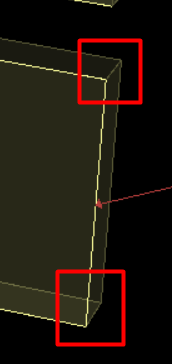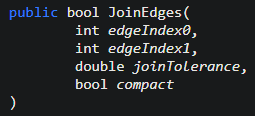# Need an example for Rhino.Geometry.Brep.JoinEdges

I’m barely familiar with RhinoCommon and there’s not a python method for this, hence the request.

Does `Rhino.Geometry.Brep.JoinNakedEdges(double tolerance)` achieve the results you need? It will save you the trouble of finding the correct indices of the edges you would like to merge.

In most cases, `brep1.Join(brep2, tolerance, True)` will do this work for you when `tolerance` is appropriately set. I’m guessing you are using this method already when joining the fin to the initial brep, so maybe you just need to increase the tolerance slightly to get rid of the naked edges.

But I don’t need to join all naked edges. That’s the whole point.
I only need the edges brown leader is pointing to joined.
Red square edges have to stay unjoined for unfolding later on:I did not realize that in your first post, sorry. My personal lazy preferred way would be to create the flaps on the side of your box in their unfolded state, and join all naked edges so you end up with the brep topology you are looking for, just in a different geometry. You can then fold/unfold it from there.

But I imagine there is a more complex case you are working on that you are not showing here, where this method would not work. In that case `JoinEdges` or `CreateFromJoinedEdges` is probably the way to go as you say.

I’m not really able to provide much help on these without understanding where you get stuck exactly. Do you have an example file that you can provide, and the code that is causing you issues? What makes you feel like the API documentation of these functions is not enough?

``````import rhinoscriptsyntax as rs

def main():

pick = rs.GetEdgeCurves("Pick surface edge or Esc/Enter to exit", 0, 1, False)
if not pick: return

edge = pick
panel = pick
refpt = pick
xplode = None

if not rs.IsSurface(panel):
xplode = rs.ExplodePolysurfaces(panel)
face = rs.PointClosestObject(refpt, xplode)
else:
face = panel

param = rs.SurfaceClosestPoint(face, refpt)
normal = rs.SurfaceNormal(face, param)
flange = rs.ExtrudeCurveStraight(edge, normal, (0, 0, 0))

if xplode: rs.DeleteObjects(xplode)
rs.DeleteObject(edge)

#Assign panel closest edge to refpt to a variable?
#Assign flange closest edge to refpt to a variable?
#join above using Rhino.Geometry.Brep.JoinEdges

if __name__ == '__main__': main()
``````

Basically I’ve no idea how to do 3 commented lines. As I said, I’m a total newb in RhinoCommon and learn as I go.How do I get Edge Index?
How do I know which edge that Edge Index is corresponding to?

– Dale

To get these edge indices, you can loop through the edges of the Brep until you find one that looks like the one you want to join. For example, from the edge that was picked, you can get the vertices at each end (in the panel brep), find the corresponding vertices in the flange brep, and then find the edge of panel that goes between these two vertices.

Here’s some code to do that. It doesn’t use rhinoscriptsyntax because I find it easier to use only one of `Rhino` or `rhinoscriptsyntax` so I don’t have to keep converting back and forth between objects and GUID.

``````import Rhino
import scriptcontext as sc

def main():
filter = Rhino.DocObjects.ObjectType.EdgeFilter
rc, objref = Rhino.Input.RhinoGet.GetOneObject(
"Select a brep edge",
False,
filter
)
if rc != Rhino.Commands.Result.Success: return rc

if not objref: return Rhino.Commands.Result.Failure

brep = objref.Brep()
edge = objref.Edge()
objref.Object().Select(False)

offset = edge.OffsetNormalToSurface(face.ToNurbsSurface(), 1.)
[edge, offset],
Rhino.Geometry.Point3d.Unset,
Rhino.Geometry.Point3d.Unset,
Rhino.Geometry.LoftType.Normal,
False
)

#brep.JoinNakedEdges(0.)

v0 = edge.StartVertex
v1 = edge.EndVertex
neigh_v0 = -1
neigh_v1 = -1
if neigh_v0 == -1 and (v0.Location - vertex.Location).IsTiny():
neigh_v0 = vertex
elif neigh_v1 == -1 and (v1.Location - vertex.Location).IsTiny():
neigh_v1 = vertex
if neigh_v0 != -1 and neigh_v1 != -1:
break
if neigh_v0 == -1 or neigh_v1 == -1:
return False

edge_to_merge = -1
for neigh_edge_idx in neigh_v0.EdgeIndices():
print(neigh_edge_idx, neigh_edge.StartVertex.VertexIndex, neigh_edge.EndVertex.VertexIndex)
if (
(
neigh_edge.StartVertex.VertexIndex == neigh_v0.VertexIndex
and neigh_edge.EndVertex.VertexIndex == neigh_v1.VertexIndex
) or (
neigh_edge.EndVertex.VertexIndex == neigh_v0.VertexIndex
and neigh_edge.StartVertex.VertexIndex == neigh_v1.VertexIndex
)
):
edge_to_merge = neigh_edge_idx
break
if edge_to_merge == -1:
return False

#brep.JoinEdges(edge.EdgeIndex, edge_to_merge, 0., False)
new_brep = Rhino.Geometry.Brep.CreateFromJoinedEdges(
brep,
edge.EdgeIndex,
edge_to_merge,
0.01
)

brep = new_brep
brep.Compact()
xf = Rhino.Geometry.Transform.Translation(10, 10, 0)
sc.doc.Objects.Transform(new_guid, xf, False)
sc.doc.Objects.Delete(sc.doc.Objects.Find(new_guid))
sc.doc.Views.Redraw()

if __name__ == "__main__":
main()

``````
1 Like

What does this line do? And what is it for?
I’m trying to deconstruct and understand what’s happening at this point.
I can’t seem to find any documentation on this.

``````    print dir (objref.Object().Select(False))
print objref.Object().Select(False)
print objref.Object().Select(True)
print objref.Object().Select(None)
``````

The documentation for all of RhinoCommon is on the Rhino developer page for APIs: https://developer.rhino3d.com/api/

`GetOneObject` returns an `ObjRef` (https://developer.rhino3d.com/api/RhinoCommon/html/M_Rhino_Input_RhinoGet_GetOneObject_1.htm), from which you can get the referenced object and de-select it (https://developer.rhino3d.com/api/RhinoCommon/html/M_Rhino_DocObjects_RhinoObject_Select.htm). It might be unnecessary but I put it there to make sure the selected edge did not stay in “selected” state after the script has run.

1 Like

I finally finished going through the whole thing, understood what each line of code is doing and I’m left with couple of questions:

Brep.CreateFromJoinedEdges creates a brand new object, so I have to delete original brep if I want to emulate JoinEdge command. Correct? Is there a way to avoid it and make new brep inherit properties of the original to truly emulate JoinEdge command?

Just call ObjectTable.Replace.

– Dale

1 Like

Makes sense! Thanks!

Now I’m stumped with this one:

I’m doing this so I can select multiple edges at once:

``````rc, objrefs = Rhino.Input.RhinoGet.GetMultipleObjects("Pick panel edge(s)", False, filter)
``````

and looping through all the single edges.

The problem is that if I pick 2 edges of the same brep, only last new_brep will be kept. I wanna say I understand why it’s happening. And I only see it being solved by replacing objref with new_brep objref, but I’ve no idea how. If at all possible…

@pierrec